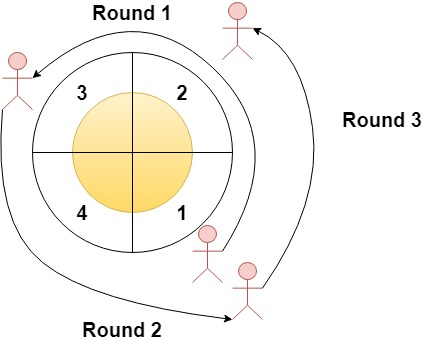Formatted question description: https://leetcode.ca/all/1560.html

# 1560. Most Visited Sector in a Circular Track (Easy)

Given an integer n and an integer array rounds. We have a circular track which consists of n sectors labeled from 1 to n. A marathon will be held on this track, the marathon consists of m rounds. The ith round starts at sector rounds[i - 1] and ends at sector rounds[i]. For example, round 1 starts at sector rounds and ends at sector rounds

Return an array of the most visited sectors sorted in ascending order.

Notice that you circulate the track in ascending order of sector numbers in the counter-clockwise direction (See the first example).

Example 1:Input: n = 4, rounds = [1,3,1,2]
Output: [1,2]
Explanation: The marathon starts at sector 1. The order of the visited sectors is as follows:
1 --> 2 --> 3 (end of round 1) --> 4 --> 1 (end of round 2) --> 2 (end of round 3 and the marathon)
We can see that both sectors 1 and 2 are visited twice and they are the most visited sectors. Sectors 3 and 4 are visited only once.

Example 2:

Input: n = 2, rounds = [2,1,2,1,2,1,2,1,2]
Output: 


Example 3:

Input: n = 7, rounds = [1,3,5,7]
Output: [1,2,3,4,5,6,7]


Constraints:

• 2 <= n <= 100
• 1 <= m <= 100
• rounds.length == m + 1
• 1 <= rounds[i] <= n
• rounds[i] != rounds[i + 1] for 0 <= i < m

Related Topics:
Array

## Solution 1.

// OJ: https://leetcode.com/problems/most-visited-sector-in-a-circular-track/

// Time: O(NM)
// Space: O(N)
class Solution {
public:
vector<int> mostVisited(int n, vector<int>& A) {
vector<int> cnt(n + 1);
int from = A;
cnt[from]++;
for (int i = 1; i < A.size(); ++i) {
int to = A[i];
while (from != to) {
++from;
if (from > n) from = 1;
cnt[from]++;
}
}
vector<int> ans;
int mx = *max_element(begin(cnt), end(cnt));
for (int i = 1; i <= n; ++i) {
if (mx == cnt[i]) ans.push_back(i);
}
return ans;
}
};


## Solution 2.

// OJ: https://leetcode.com/problems/most-visited-sector-in-a-circular-track/

// Time: O(N)
// Space: O(1)
// Ref: https://leetcode.com/problems/most-visited-sector-in-a-circular-track/discuss/806814/JavaC%2B%2BPython-From-Start-to-End
class Solution {
public:
vector<int> mostVisited(int n, vector<int>& A) {
vector<int> ans;
if (A <= A.back()) {
for (int i = A; i <= A.back(); ++i) ans.push_back(i);
} else {
for (int i = 1; i <= A.back(); ++i) ans.push_back(i);
for (int i = A; i <= n; ++i) ans.push_back(i);
}
return ans;
}
};


Java

class Solution {
public List<Integer> mostVisited(int n, int[] rounds) {
int[] visits = new int[n];
visits[rounds - 1]++;
int maxVisit = 1;
int roundsCount = rounds.length;
for (int i = 1; i < roundsCount; i++) {
int start = rounds[i - 1] - 1, end = rounds[i] - 1;
int curLength = (end - start + n) % n;
int index = start;
for (int j = 0; j < curLength; j++) {
index = (index + 1) % n;
visits[index]++;
maxVisit = Math.max(maxVisit, visits[index]);
}
}
List<Integer> list = new ArrayList<Integer>();
for (int i = 0; i < n; i++) {
if (visits[i] == maxVisit)